# Ex 1: Graph a Quadratic Inequality on the Coordinate Plane

##By Mathispower4u

Ex 1: Graph a Quadratic Inequality on the Coordinate Plane# Quarter 2, Lesson 1: Coordinate Plane, Introduction to Graphing Linear Equations

##By Stan Lisle

In this video clip, the lesson reviews the coordinate planes and prepares the viewers to start graphing equations. The video provides clear examples with questions for viewers to answer after each lesson.# Graphing Lines on the Coordinate Plane

##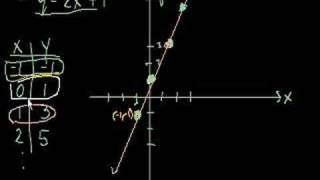By Khan Academy

This video lecture series on Algebra from Khan Academy includes Linear Equations Solving Inequalities Slope and Y-intercept Equation of a Line Averages and Integer sums.# Parallelogram on the coordinate plane

##By Khan Academy

Remember our discussion of the coordinate plane? Sure you do! Let's graph the given coordinates of three of the polygon vertices and find where the 4th vertex is.# Coordinate plane: graphing points word problem

##By Khan Academy

Naomi needs some help counting the number of blocks she walks from home to school. We'll use the coordinate plane to graph it out first. Let's go.# Coordinate plane word problems in the first quadrant

##By Khan Academy

Naomi needs some help counting the number of blocks she walks from home to school. We'll use the coordinate plane to graph it out first. Let's go.# Dilation Of Objects On The Coordinate Plane

##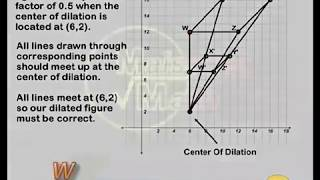By mrmaisonet

Dilation Of Objects On The Coordinate Plane# Algebra Basics: Graphing On The Coordinate Plane - Math Antics

##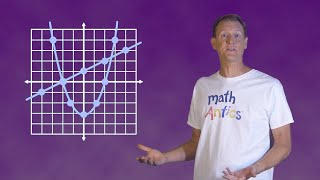By mathantics# Geometry: 7-6 Dilations and Similarity in the Coordinate Plane

##By Sir Tyler Tarver

How to apply similarity properties in the coordinate plane and use a coordinate proof to prove figures similar. Yeah yeah.# Graphing points

##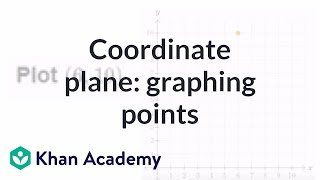By Khan Academy

This exercise asks us to graph a set of points from an ordered pair on the x and y axis of a coordinate plane.# Find inverse values of functions

##By Khan Academy

Sal is given a line segment on the coordinate plane, and he graphs the inverse of the function represented by that segment.# Find inverse values of functions

##By Khan Academy

Sal is given a line segment on the coordinate plane, and he graphs the inverse of the function represented by that segment.# Find inverse values of functions

##By Khan Academy

Sal is given a line segment on the coordinate plane, and he graphs the inverse of the function represented by that segment.# Find inverse values of functions

##By Khan Academy

Sal is given a line segment on the coordinate plane, and he graphs the inverse of the function represented by that segment.# Find inverse values of functions

##By Khan Academy

Sal is given a line segment on the coordinate plane, and he graphs the inverse of the function represented by that segment.# Graphing points

##By Khan Academy

This exercise asks us to graph a set of points from an ordered pair on the x and y axis of a coordinate plane.# Transformations - Translating A Triangle On The Coordinate Plane

##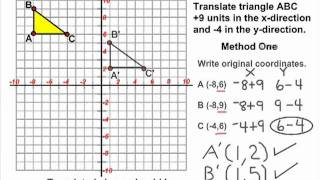By mrmaisonet

This tutorial reviews how to perform a translation on the coordinate plane using a triangle.# Coordinate plane: graphing points and naming quadrants

##By Khan Academy

This is a great exercise example in which we plot the ordered pair and then identify which quadrant the point lies. You'll get the hang of this quickly!# Finding the Distance Between Two Points on a Coordinate Plane

##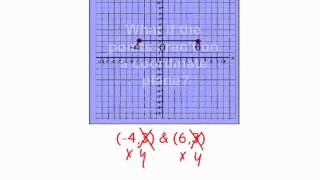By SmithMathAcademy

(+) Calculate the distance between numbers in the complex plane as the modulus of the difference, and the midpoint of a segment as the average of the numbers at its endpoints.# Geometry problems on the coordinate plane

##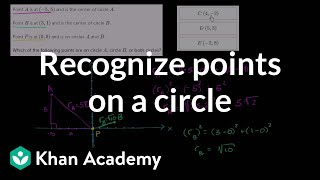By Khan Academy

Watch Sal solve a challenging problem where he has to determine if points are on both, one, or neither of two circles.